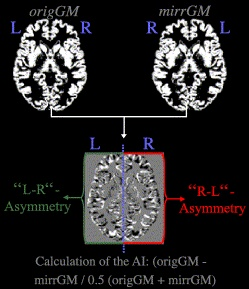## Cross-sectional data asymmetry

In order to explore lateralization effects you have to process your raw images using a symmetrical template. After creating customized templates you can use the function “Cross-sectional data asymmetry: calculate lateralization index” from the “Segment” menu to calculate lateralization index of your segmentations. The GM template will be internally averaged with its mirrored image to obtain a symmetrical template indicated at the end of the filename by the term “_sym”. This symmetric template will be automatically created and saved in a temporary folder and will be used to segment data and to calculate lateralization index (LI) using the following formula:

LI = 2*(R-L)/(R+L)

“R” means that the original images is used and “L” represents the mirrored image. The LI files are indicated by the prefix “LI_” and can be smoothed and used in the statistical analysis.Calculation of the lateralization index (=asymmetry index).

The interpretation of the LI values is as follows:
LI > 0 in left hemisphere or LI < 0 in right hemisphere: more leftward asymmetry (left > right)
LI < 0 in left hemisphere or LI > 0 in right hemisphere: more rightward asymmetry (right > left)

Keep in mind that you now also have negative values and the usual use of an absolute threshold of > 0 would excludes all negative values. I recommend to use an absolute threshold of “-Inf” and to use an implicite and/or user defined explicite mask.
This approach was used in the paper of Luders et al. (2004).